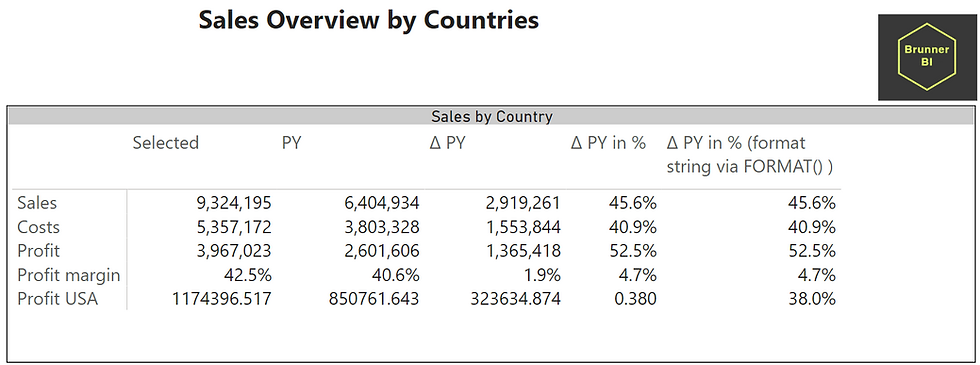top of page
Suche

# Format strings for calculation groups in live reports

According to sqlbi, it is advised to create format string expressions for calculation groups in the respective "Options" field in Tabular Editor.

If you start adding new measures in live reports however, the format string might not work as expected.

This is the scenario:

1) We have a dataset and are building a report on it via live connection.

2) This dataset contains a calculation group.

3) If we now add a new measure in the live report, the format string expression will not work properly as seen below. (Profit USA is a measure created in the live report)How do we fix this?

Instead of defining the format string expression here (this is in Tabular Editor):We need to leave Format String Expression empty and rather apply the format using DAX code.After having done it this way, even measures created in the live report will have the correct look, as seen below in the last column on the right.

There is one downside though, using FORMAT() we convert the value to text, thus we cannot use this for charts e.g. anymore.You can see the difference between the last and the second last column (for measure Profit USA)

These two calculation items only differ in where the format string expression is applied.

The last column/calculation item (where it works correctly) has this content and has an empty format string expression in Tabular Editor.

```VAR TY =
SELECTEDMEASURE ()
VAR PY =
CALCULATE ( SELECTEDMEASURE (), DATEADD ( 'Calendar'[Date], -1, YEAR ) )
RETURN
FORMAT ( DIVIDE ( TY - PY, ABS ( PY ) ), "#,0.0%;-#,0.0%;#,0.0%
" )```

The second last column however (or calculation item) holds this code and has the format string expression applied in the "Options" field in Tabular Editor.

```VAR TY =
SELECTEDMEASURE ()
VAR PY =
CALCULATE ( SELECTEDMEASURE (), DATEADD ( 'Calendar'[Date], -1, YEAR ) )
RETURN
DIVIDE ( TY - PY, ABS ( PY ) )```

If you have faced this or a similar issue or you would like to reach out to us, feel free to comment.

305 Ansichten0 Kommentare

Alle ansehen
bottom of page